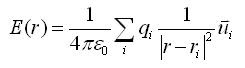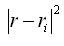### Electric Fields, Charge Distributions, and the GRE

Reviewing for the GRE physics subject test, I've frequently come across questions like:

Given a distribution of discrete charges, (as shown in the diagram), determine the electric field at a given point r, (also shown in the diagram).Formula for the Electric Field from a Distribution of Charges
The distribution of charges I've seen in sample questions have, so far, involved only finite numbers of point charges, so the electric field equation can be written as a sum:whereis the distance from a charge contributing to the sum to the location where the electric field is being measured, q is the value of the particular charge, and u is the unit vector pointing from the contributing charge to the field measurement point.

This can be separated into x and y components:where theta is the angle between the particular charge and the point where the field is being measured with respect to the x axis. Using these formulas, it's just a matter of summing up the contribution of each point charge to the electric field at the location defined by the problem.

So, the standard method is to determine the x and y contribution from each charge at the location specified by the problem, and then simply sum up all the contributions. Let's try one out:

Example

First, we'll try the example from the diagram above:The contributions from each charge are shown in the table below:

Here's one more example with three equal charges space equally around the unit circle:The angles used to calculate the x and y contributions of each field are also shown.

The contributions from each charge are shown in the table below:

The Trick
Both of these examples also point out a type of 'trick' question that appears in the samples. When a set of equal charges is evenly spaced around a circle surrounding the field measurement point, the total field is 0. Watch for this pattern.

Handy Stuff
GRE Physics Sample Test [pdf]
Other links to GRE sample questions

### Cool Math Tricks: Deriving the Divergence, (Del or Nabla) into New (Cylindrical) Coordinate Systems

Now available as a Kindle ebook for 99 cents! Get a spiffy ebook, and fund more physics
The following is a pretty lengthy procedure, but converting the divergence, (nabla, del) operator between coordinate systems comes up pretty often. While there are tables for converting between common coordinate systems, there seem to be fewer explanations of the procedure for deriving the conversion, so here goes!

What do we actually want?

To convert the Cartesian nabla

to the nabla for another coordinate system, say… cylindrical coordinates.

What we’ll need:

1. The Cartesian Nabla:

2. A set of equations relating the Cartesian coordinates to cylindrical coordinates:

3. A set of equations relating the Cartesian basis vectors to the basis vectors of the new coordinate system:

How to do it:

Use the chain rule for differentiation to convert the derivatives with respect to the Cartesian variables to derivatives with respect to the cylindrical variables.

The chain rule can be used to convert a differe…

### Division: Distributing the Work

Our unschooling math comes in bits and pieces.  The oldest kid here, seven year-old No. 1 loves math problems, so math moves along pretty fast for her.  Here’s how she arrived at the distributive property recently.  Tldr; it came about only because she needed it.
“Give me a math problem!” No. 1 asked Mom-person.

“OK, what’s 18 divided by 2?  But, you’re going to have to do it as you walk.  You and Dad need to head out.”

And so, No. 1 and I found ourselves headed out on our mini-adventure with a new math problem to discuss.

One looked at the ceiling of the library lost in thought as we walked.  She glanced down at her fingers for a moment.  “Is it six?”

“I don’t know, let’s see,” I hedged.  “What’s two times six?  Is it eighteen?”

One looked at me hopefully heading back into her mental math.

I needed to visit the restroom before we left, so I hurried her calculation along.  “What’s two times five?”

I got a grin, and another look indicating she was thinking about that one.

I flashed eac…